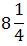# If x/2y = 6/7, the value ofis:

1.  13/19

2.  15/19

3.  1

4.

4

1

Explanation :
No Explanation available for this question

# Ifis:

1.  1/7

2.  7/25

3.  3/4

4.  None of these

4

7/25

Explanation :
No Explanation available for this question

# If (a –b) is 6 more than (c + d) and (a + b) is 3 less than (c – d), then (a – c) is:

1.  0.5

2.  1

3.  1.5

4.  None of these

4

1.5

Explanation :
No Explanation available for this question

# If x = a/a-1 and y =1/a – 1, then :

1.  x is equal to y

2.  x is equal to y only if a< 1

3.  x is greater than y

4.  x is greater than y only  if a< 1

5.  y is greater than x only if a

5

x is greater than y

Explanation :
No Explanation available for this question

# If 0 < a< 1, then the value of a + 1/a is:

1.  Less than 2

2.  greater than 2

3.  less than 4

4.  greater than 4

4

greater than 2

Explanation :
No Explanation available for this question

# If a/x + y/b = 1 and b/y + z/c= 1, then x/a + c/z will be equal to:

1.  0

2.  b/y

3.  1

4.  y/b

4

1

Explanation :
No Explanation available for this question

# If a, b, c are integers; a2 + b2 = 45 and b2 +c2 = 40, then the values of a, b and c respectively are:

1.  2, 6, 3

2.  3, 2, 6

3.  5, 4, 3

4.  None of these

4

3, 2, 6

Explanation :
No Explanation available for this question

# If 3x + 7 = x2 + P – 7x + 5, what is the value of P

1.  1/2

2.

3.

4.  Cannot be determined

4Explanation :
No Explanation available for this question

# If 2a + b/a + 4b =3, then find the value of a + b/a + 2b.

1.  2/7

2.  5/9

3.  10/7

4.  10/9

4

10/9

Explanation :
No Explanation available for this question

1.  3/8

2.  19/8

3.  8/3

4.  8/19

4